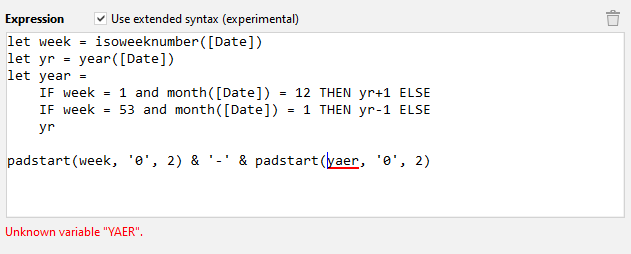# 💡 Announcement: Extended expression syntax

We’re working on a new, extended expression syntax in v4.4 that is planned for release by the end of May. The changes:

### Unary minus operator

It will be possible to use the unary minus. So instead of writing

``````addmonths([Date], 0 - 1)
``````

it will be possible to write

``````addmonths([Date], -1)
``````

Long due. I know### Local constants

We’re introducing constants in expressions. Constants must be assigned using the `let` operator before they can be used in expression. Syntax:

``````//Constant assignment.
let a = ([A] + [B]) / [C]       // Some complex expression

//Use of constant in expression.
([D] + a) / ([E] - a)
``````

Constants should help make expressions more readable. They also eliminate the need to create temporary columns in some cases.

### IF/THEN/ELSE operator

Since the very early versions EasyMorph has been offering the if() function which works similarly to the IF() function in Excel.

Now, for better readability of expressions we’re adding the IF/THEN/ELSE operator, which is well known for Tableau users. Example:

``````IF isempty([FX Rate]) THEN error("Missing FX rate!") ELSE [Amount] * [FX Rate]
``````

The ELSE statement is optional. If omitted it returns an empty value.

``````//If FX rate is empty the result will be empty too.
IF not isempty([FX Rate]) THEN [Amount] * [FX Rate]
``````

Question to our users: how do these improvements look to you?

I have business users so constants in “let” expression is a bit complex for them. Maybe it could be good to have a third tab : columns, parameters AND variables, even if it’s only for the current expression. You would be able to define variables there, so no “let” syntax and you can drag and drop like columns/parameters.

For unary minus, it was so much expected, thank you !!!

The IF syntax on the contrary of “let” is better for business users, clearer than coder syntax with “(” and “;”. Well that’s our point of view. It’s good !

Here is a practical example. Which reads better?

This:

``````padstart(isoweeknumber([Date]), '0', 2) & '-' & padstart(if(isoweeknumber([Date]) = 1 and month([Date]) = 12, year([Date])+1, year([Date])), '0', 2)
``````

Or this:

``````let week = isoweeknumber([Date])
let yr = year([Date])

//attribute the last incomplete week to the next year
let year = (IF week = 1 and month([Date]) = 12 THEN yr+1 ELSE yr)

``````
1 Like

Hi Dmitry,

Very good improvements ! Will existing projects that contain the old syntax will continue to run correctly after the update?

Maybe the “let” keyword is not that clear. Personally I would prefer “var” but I consider it a detail. I know that javascript also uses the “let” keyword.

Any plans to introduce some syntax highlighting, autocompletion and auto-indentation in the expression builder as expressions will become more complex due to the new possibilities?

Thanks !
Nikolaas

This will appear at some point, but not in the next few releases. The only addition in the next release will be highlighting of syntax errors.

Yes, the new syntax is an extension of the old one. Expressions in old projects will keep working without any changes.

Geat !

Here is what error highlighting will look like.1 Like